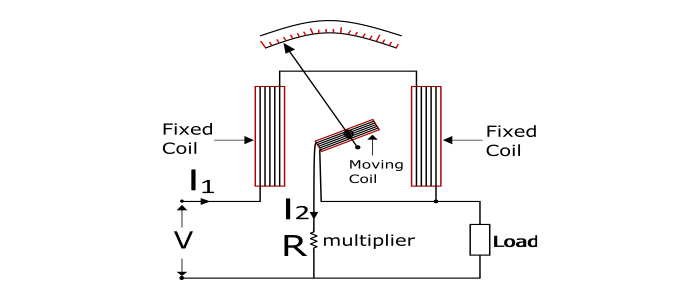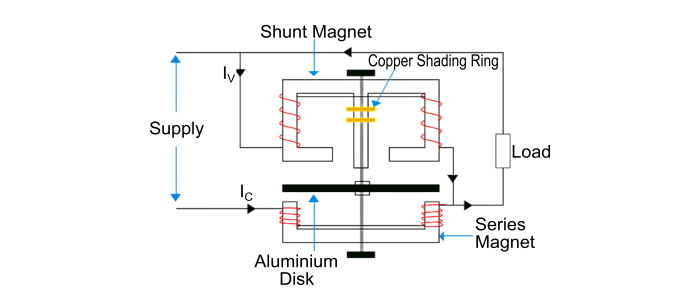# Wattmeter â€“ Types and Working Principle

A wattmeter is an instrument which is used to measure electric power given to or developed by an electrical circuit. Generally, a wattmeter consists of a current coil and a potential coil.

## Types of Wattmeter

• Electrodynamometer wattmeter – for both DC and AC power measurement

• Induction wattmeter – for AC power measurement only

## Working Principle of Electrodynamometer Wattmeter

The electrodynamometer wattmeter works on the dynamometer principle i.e. a mechanical force acts between two current carrying conductors or coils.

It consists of a fixed which is divided into two halves which are parallel to each other and is connected in series with the load while the moving is connected across the load through a series multiplier resistance. The fixed coil is called current coil while the moving coil is called potential coil.When the wattmeter is connected in a circuit to measure power. The current coil carries the load current and potential coil carries a current proportional to the load voltage. Due to the currents in the coils, a mechanical force acts between them. The result of this mechanical force deflects the moving coil and the pointer over the scale. The pointer comes to the rest when the deflecting torque and controlling torque become equal. As the reversal of the circuit current reverses the currents in both the current coil and potential coil so that the direction of deflecting torque remains unchanged. Therefore, electrodynamometer wattmeter can measure power in both the AC as well DC circuits.

## Working Principle of Induction Wattmeter

The induction type wattmeter can be used to measure AC power only.

The working of induction type wattmeter is based on the principle of electromagnetic induction.

The induction wattmeter consists of two laminated electromagnets viz. Shunt Magnet and Series Magnet. The shunt magnet is connected across the supply and carries a current proportional to the supply voltage. The coil of shunt magnet is made highly inductive so that the current in it lags the supply voltage by 90°. The series magnet is connected in series with the supply and carries the load current. The coil of series magnet is made highly noninductive. A thin disc (made up of aluminium) mounted on a spindle is placed between the two magnets so that it cuts the flux of the two magnets.When the wattmeter is connected in an AC circuit, a current flows through the coil of the shunt magnet that is proportional to the supply voltage and the series magnet carries the load current. The fluxes produced by the two magnets induce eddy currents in the aluminium disc by the action of electromagnetic induction. Due to the interaction between the fluxes and eddy currents, a deflecting torque is produced on the disc, causing the disc to move and hence, the pointer connected to the disc moves over the scale. The pointer comes to rest when the deflecting torque becomes equal to the controlling torque.